Objectives:

• To understand the terms reflection and refraction of all wavesWhen we look in a mirror, why is it that the image we see is as we expect? Why is it not deformed or bent in some way?

Reflection

This area of Physics is frequently considered to be ‘easy’ and ‘straightforward’, this is because we are familiar with what we see in the mirror – the image seen (often) as we expect; and we use mirrors daily.

“Light travels in straight lines” – this is a statement you will be familiar with since the KS3. Well if we use this and apply it to a light box emitting light through a narrow slit directed at a mirror, we can experiment to determine why the image we see in a mirror ir always the same and as we expect.A ray box directing a narrow beam of light towards to mirror will reflect the light off it. There the incident initial) ray strikes the mirror, a normal line needs to be drawn (the normal line is a perpendicular line to the mirror). The angle between the normal and the incident ray can then be measured using a protractor.

The reflected ray will be visible (depending on the lighting of the rest of the room you are in). These rays should be marked so accurate angles can be measure, as shown;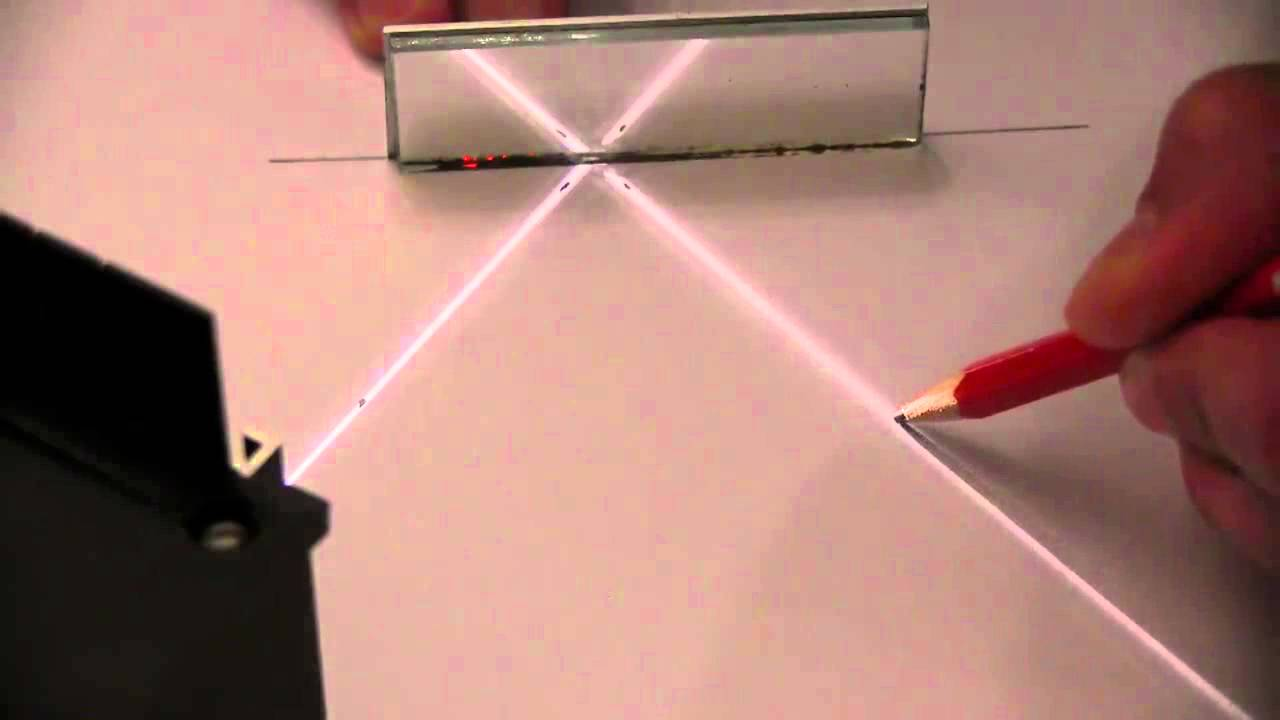Using a protractor then, the angle of incidence can be measure. The angle at which the ray is reflected can also be measured – this is the angle from the normal to the reflect ray.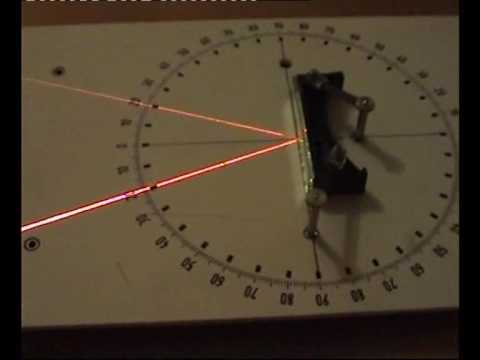It can be observed that when carried out with a narrow beam of light and with accurate measurements, that the angle of incidence is always equal to the angle of reflection.$i = r$

This equation holds true for light reflecting off any surface. It is because the angle of incidence is equal to the angle of reflection that overtime we look into a mirror we should see our normal image in front of us. If$i \neq r$ , our image in mirror would not look as we expect.

The following is the type of diagram that we draw for reflection;If a surface is a rough surface, the equation$i = r$  so holds true except the surface will have jagged edges in all directions, which means the angle of incidence varies and thus the angle of reflection will also vary.

Refraction

Refraction is another term used to describe the the change in direction that light may undergo when travelling. It differs from reflection in that the light will pass through from one transmission medium to another. If the object changes direction during this process it is referred to as refraction. This type of occurrence happens all around us everyday, like when you see objects at the bottom of a swimming pool or through a window;As light travels from one medium to another it can change direction. This is because it either speeds up or slows down.

Light travels its fastest in a vacuum, as it enters a different material, even out atmosphere, it slows down. It is this change in speed that causes a change in direction.

When drawing refracted rays we stick to the same style of diagrams as we draw for reflection, the angles are always drawn from the normal to the relevant ray;The following are some laws by which light sticks to when undergoing refraction;

1. The incident ray, the refracted ray and the normal are all in the same plane.
2. The ratio of the sine of the angle of incidence to the sine of the angle of refraction is the same for all rays travelling between a given boundary – this is known as Snell’s law;$\frac{\sin(i)}{\sin(r)} = constant$

When we see objects it is usually because the light travels through/ off it and changes direction. It is this change in direction (and hence refraction) that gives us the shape of the object and allows us to see it.

Light gets refracted when passing through glass blocks, you may do an experiment in class to demonstrate this affect;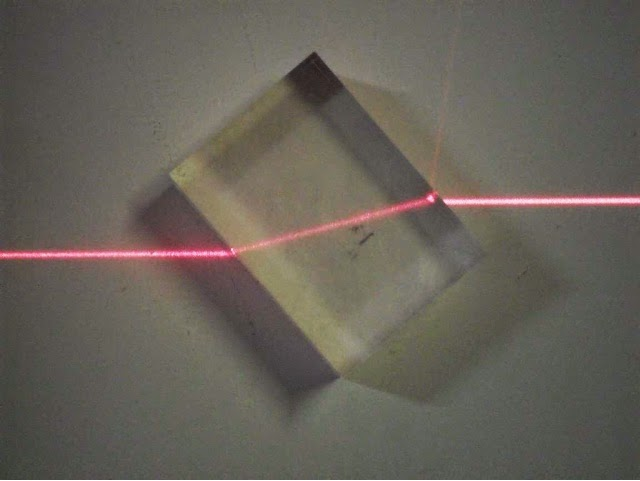Light also refracts when passing through our glass windows, we don’t often notice it because the amount that light refracts going into the object is the same as that when leaving the object.

There are lots of cool effects you can demonstrate refraction with, the following images show some – they range from things you would notice on a day-to-day basis to more impressive scenarios;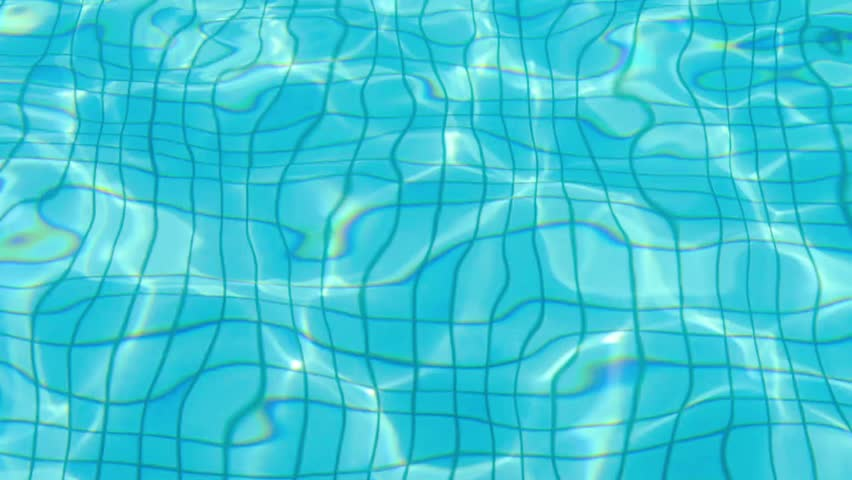Tiles on the bottom of a swimming pool, they are actually square but the waves on the surface are refracting light differently through the water.Straws appear to be snapped or bent, but actually it’s due to the curvature of the glass and the liquid inside.The striped background is still striped, but the liquid in the glass has changed the direction of light through it affectively making the striped appear skewed.This white tiger does not actually have an overly large body, this is the water causing the light to refract.This polar bear has not been decapitated, it is the light being forced to refract through the different mediums, air and water.These are water droplets in front of what I can only assume is an image of Jupiter. Look at the lower droplet it appears to show a mini Jupiter inside of it.This is an image of the Sunset. If you notice though, the sky appears both above and below this section of the sun that has become visible. This means that the top of the sun has actually been refracted due to the atmosphere such that it is visible.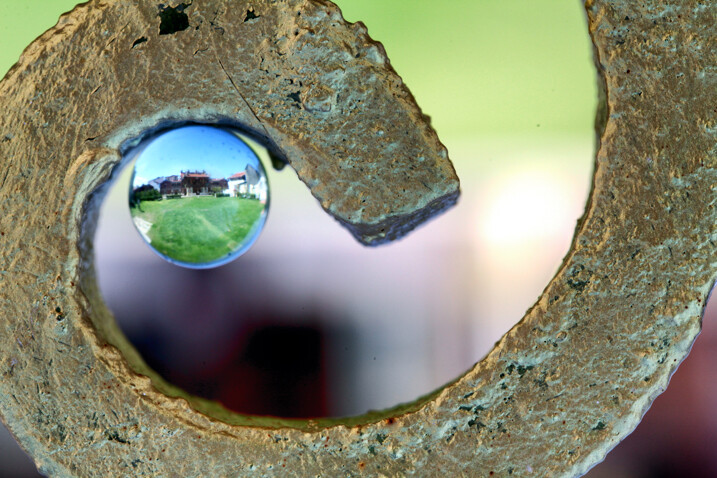Another water droplet showing an image of a garden in it – giving a hint to what is beyond the droplet itself.

White Light

Refraction can be used to show the true make up of white light. When shone, as a beam through a prism, white light gets refracted and exits in the form of a spectrum of colours;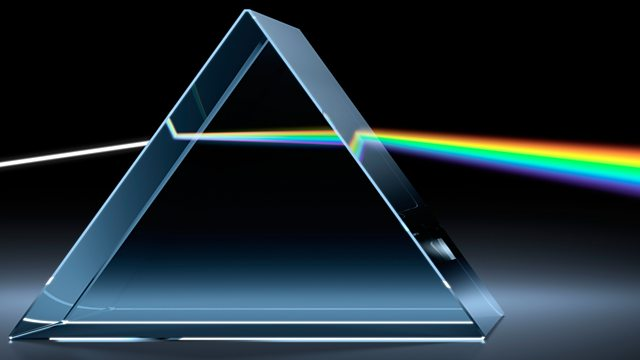The white light enters, gets refracted and then when exiting the prism gets refracted again.

The colours of the rainbow are seen to exit instead of the white beam.

The following diagram helps to show this in more a little more clearly;Each colour has a different frequency from the others. It is the frequency of light that ultimately determines what colour it is, white light is a mixture of all the colours merged together and the sun emits its visible light as white light.

Factors affecting refraction

• The fact that the different colours refract by different amount suggests that the amount a ray refracts is dependent on the frequency.
• It was also mentioned previously that the speed at which light travels (for the change in speed between two mediums) affects the amount that the light refracts

Refractive index is a term used to describe how light travels through a medium, it is defined as;$n = \frac{c}{v}$

where;$n$   is the refractive index, it is a dimensionless number$v$  is the speed of light in that medium, measured in$ms^{-1}$$c$ is the speed of light in a vacuum, measured in$ms^{-1}$

So the speed ultimately determines the refractive index of the light and hence how much the light changes direction in a medium. How does frequency play a part? If you think back to the wave speed equation;$c = f \lambda$ . Frequency is the quantity that defines how much energy a ‘ray’ of light has, the amount of energy it has determines the colour we see of that ‘ray’, it is a fixed quantity for any particular light ‘ray’. Using this and the equation for speed above, if the speed of light changes because it enters a new medium and the frequency is unchanged the wavelength must change (if the speed decreases then the wavelength increases).

In a vacuum all light travel at the speed of light,$3 \times 10^{8} ms^{-1}$ , this means that the wavelength of each colour is a certain length (or a small range in lengths). When the light enters a new medium and slows down the frequency remains constant (and the same value as it would be in a vacuum) and the wavelength increases.

It is not the wavelength that determines how much light refracts in a new medium because it changes as it goes into a new medium. The frequency does not change and hence is the property that is referred to when discussing refraction.